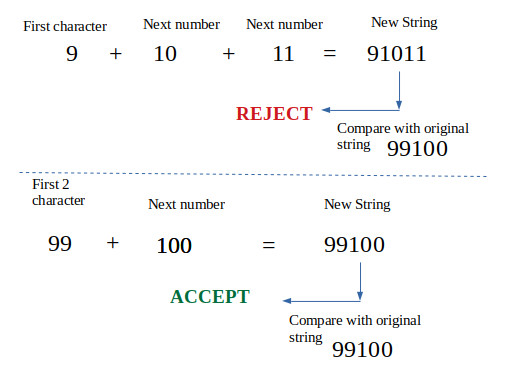GeeksforGeeks App
Open AppBrowser
Continue

# Consecutive sequenced numbers in a string

Given a string that contains only numeric digits, we need to check whether that strings contains numbers in a consecutive sequential manner in increasing order.

Note: Negative numbers are not considered part of this problem. So we consider that input only contains positive integer.

Examples:

```Input :  str = "1234"
Output : Yes
1
Explanation :
There are 1, 2, 3, 4 which are
consecutive and in increasing order.
And the starting number is 1

Input :  str = "91012"
Output : No
Explanation :
There are no such sequence in the
string.

Input :  str = "99100"
Output : Yes
99
Explanation : The consecutive sequential
numbers are 99, 100

Input :  str = "010203"
Output : NO
Explanation :
Although at first glance there seems to
be 01, 02, 03. But those wouldn't be
considered a number. 01 is not 1  it's 0, 1 ```

Approach: An easily implementable and useful approach is to start taking one character at first (assuming that our string starts with 1 digit number) and then form a new string by concatenating the next number until the length of new string is equal to original string.

Perhaps an example may clarify :

Let’s take string “99100”Below is the implementation:

## C++

 `// CPP Program to check whether a string contains``// consecutive sequential numbers or not``#include ``using` `namespace` `std;` `// function to check consecutive sequential number``int` `isConsecutive(string str)``{``    ``// variable to store starting number``    ``int` `start;` `    ``// length of the input string``    ``int` `length = str.size();` `    ``// find the number till half of the string``    ``for` `(``int` `i = 0; i < length / 2; i++) {` `        ``// new string containing the starting``        ``// substring of input string``        ``string new_str = str.substr(0, i + 1);` `        ``// converting starting substring into number``        ``int` `num = ``atoi``(new_str.c_str());` `        ``// backing up the starting number in start``        ``start = num;` `        ``// while loop until the new_string is``        ``// smaller than input string``        ``while` `(new_str.size() < length) {` `            ``// next number``            ``num++;` `            ``// concatenate the next number``            ``new_str += to_string(num);``        ``}` `        ``// check if new string becomes equal to``        ``// input string``        ``if` `(new_str == str)``           ``return` `start;``    ``}` `    ``// if string doesn't contains consecutive numbers``    ``return` `-1;``}` `// Driver's Code``int` `main()``{``    ``string str = ``"99100"``;``    ``cout << ``"String: "` `<< str << endl;``    ``int` `start = isConsecutive(str);``    ``if` `(start != -1)``        ``cout << ``"Yes \n"` `<< start << endl;``    ``else``        ``cout << ``"No"` `<< endl;` `    ``string str1 = ``"121315"``;``    ``cout << ``"\nString: "` `<< str1 << endl;``    ``start = isConsecutive(str1);``    ``if` `(start != -1)``        ``cout << ``"Yes \n"` `<< start << endl;``    ``else``        ``cout << ``"No"` `<< endl;` `    ``return` `0;``}`

## Java

 `// Java Program to check whether a String contains``// consecutive sequential numbers or not``class` `GFG``{` `// function to check consecutive sequential number``static` `int` `isConsecutive(String str)``{``    ``// variable to store starting number``    ``int` `start;` `    ``// length of the input String``    ``int` `length = str.length();` `    ``// find the number till half of the String``    ``for` `(``int` `i = ``0``; i < length / ``2``; i++)``    ``{` `        ``// new String containing the starting``        ``// substring of input String``        ``String new_str = str.substring(``0``, i + ``1``);` `        ``// converting starting substring into number``        ``int` `num = Integer.parseInt(new_str);` `        ``// backing up the starting number in start``        ``start = num;` `        ``// while loop until the new_String is``        ``// smaller than input String``        ``while` `(new_str.length() < length)``        ``{` `            ``// next number``            ``num++;` `            ``// concatenate the next number``            ``new_str = new_str + String.valueOf(num);``        ``}` `        ``// check if new String becomes equal to``        ``// input String``        ``if` `(new_str.equals(str))``        ``return` `start;``    ``}` `    ``// if String doesn't contains consecutive numbers``    ``return` `-``1``;``}` `// Driver Code``public` `static` `void` `main(String[] args)``{``    ``String str = ``"99100"``;``    ``System.out.println(``"String: "` `+ str);``    ``int` `start = isConsecutive(str);``    ``if` `(start != -``1``)``        ``System.out.println(``"Yes \n"` `+ start);``    ``else``        ``System.out.println(``"No"``);` `    ``String str1 = ``"121315"``;``    ``System.out.println(``"\nString: "` `+ str1);``    ``start = isConsecutive(str1);``    ``if` `(start != -``1``)``        ``System.out.println(``"Yes \n"` `+ start);``    ``else``        ``System.out.println(``"No"``);``    ``}``}` `// This code contributed by Rajput-Ji`

## Python3

 `# Python Program to check whether a String contains``# consecutive sequential numbers or not` `# function to check consecutive sequential number``def` `isConsecutive(strs):``  ` `    ``# variable to store starting number``    ``start ``=` `0``;` `    ``# length of the input String``    ``length ``=` `len``(strs);` `    ``# find the number till half of the String``    ``for` `i ``in` `range``(length ``/``/` `2``):` `        ``# new String containing the starting``        ``# substring of input String``        ``new_str ``=` `strs[``0``: i ``+` `1``];` `        ``# converting starting substring into number``        ``num ``=` `int``(new_str);` `        ``# backing up the starting number in start``        ``start ``=` `num;` `        ``# while loop until the new_String is``        ``# smaller than input String``        ``while` `(``len``(new_str) < length):``            ``# next number``            ``num ``+``=` `1``;` `            ``# concatenate the next number``            ``new_str ``=` `new_str ``+` `str``(num);` `        ``# check if new String becomes equal to``        ``# input String``        ``if` `(new_str``=``=``(strs)):``            ``return` `start;` `    ``# if String doesn't contains consecutive numbers``    ``return` `-``1``;` `# Driver Code``if` `__name__ ``=``=` `'__main__'``:``    ``str0 ``=` `"99100"``;``    ``print``(``"String: "` `+` `str0);``    ``start ``=` `isConsecutive(str0);``    ``if` `(start !``=` `-``1``):``        ``print``(``"Yes \n"` `, start);``    ``else``:``        ``print``(``"No"``);` `    ``str1 ``=` `"121315"``;``    ``print``(``"\nString: "` `, str1);``    ``start ``=` `isConsecutive(str1);``    ``if` `(start !``=` `-``1``):``        ``print``(``"Yes \n"` `, start);``    ``else``:``        ``print``(``"No"``);` `# This code is contributed by shikhasingrajput`

## C#

 `// C# Program to check whether a String contains``// consecutive sequential numbers or not``using` `System;` `class` `GFG``{` `// function to check consecutive sequential number``static` `int` `isConsecutive(String str)``{``    ``// variable to store starting number``    ``int` `start;` `    ``// length of the input String``    ``int` `length = str.Length;` `    ``// find the number till half of the String``    ``for` `(``int` `i = 0; i < length / 2; i++)``    ``{` `        ``// new String containing the starting``        ``// substring of input String``        ``String new_str = str.Substring(0, i + 1);` `        ``// converting starting substring into number``        ``int` `num = ``int``.Parse(new_str);` `        ``// backing up the starting number in start``        ``start = num;` `        ``// while loop until the new_String is``        ``// smaller than input String``        ``while` `(new_str.Length < length)``        ``{` `            ``// next number``            ``num++;` `            ``// concatenate the next number``            ``new_str = new_str + String.Join(``""``,num);``        ``}` `        ``// check if new String becomes equal to``        ``// input String``        ``if` `(new_str.Equals(str))``        ``return` `start;``    ``}` `    ``// if String doesn't contains consecutive numbers``    ``return` `-1;``}` `// Driver Code``public` `static` `void` `Main(String[] args)``{``    ``String str = ``"99100"``;``    ``Console.WriteLine(``"String: "` `+ str);``    ``int` `start = isConsecutive(str);``    ``if` `(start != -1)``        ``Console.WriteLine(``"Yes \n"` `+ start);``    ``else``        ``Console.WriteLine(``"No"``);` `    ``String str1 = ``"121315"``;``    ``Console.WriteLine(``"\nString: "` `+ str1);``    ``start = isConsecutive(str1);``    ``if` `(start != -1)``        ``Console.WriteLine(``"Yes \n"` `+ start);``    ``else``        ``Console.WriteLine(``"No"``);``    ``}``}` `// This code has been contributed by 29AjayKumar`

## Javascript

 ``

Output

```String: 99100
Yes
99

String: 121315
No```

Time Complexity: O(n2), where n is the size of the given string.
Auxiliary Space: O(n), where n is the size of the given string.

My Personal Notes arrow_drop_up